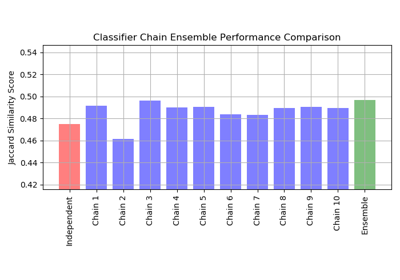# sklearn.metrics.jaccard_similarity_score¶

sklearn.metrics.jaccard_similarity_score(y_true, y_pred, normalize=True, sample_weight=None)[source]

Jaccard similarity coefficient score

The Jaccard index , or Jaccard similarity coefficient, defined as the size of the intersection divided by the size of the union of two label sets, is used to compare set of predicted labels for a sample to the corresponding set of labels in y_true.

Read more in the User Guide.

Parameters: y_true : 1d array-like, or label indicator array / sparse matrix Ground truth (correct) labels. y_pred : 1d array-like, or label indicator array / sparse matrix Predicted labels, as returned by a classifier. normalize : bool, optional (default=True) If False, return the sum of the Jaccard similarity coefficient over the sample set. Otherwise, return the average of Jaccard similarity coefficient. sample_weight : array-like of shape = [n_samples], optional Sample weights. score : float If normalize == True, return the average Jaccard similarity coefficient, else it returns the sum of the Jaccard similarity coefficient over the sample set. The best performance is 1 with normalize == True and the number of samples with normalize == False.

Notes

In binary and multiclass classification, this function is equivalent to the accuracy_score. It differs in the multilabel classification problem.

References

Examples

>>> import numpy as np
>>> from sklearn.metrics import jaccard_similarity_score
>>> y_pred = [0, 2, 1, 3]
>>> y_true = [0, 1, 2, 3]
>>> jaccard_similarity_score(y_true, y_pred)
0.5
>>> jaccard_similarity_score(y_true, y_pred, normalize=False)
2


In the multilabel case with binary label indicators:

>>> jaccard_similarity_score(np.array([[0, 1], [1, 1]]),        np.ones((2, 2)))
0.75


## Examples using sklearn.metrics.jaccard_similarity_score¶Classifier Chain